Blank (_), BlankSequence (__), BlankNullSequence (___)

Blank (_), BlankSequence (__) and BlankNullSequence (___) are the most primative of Mathematica's pattern matching features.

Basic Blank (_) usage

In the first example Blank (_) is used for individual arguments.  In this case (f) is only defined when it's given two arguments.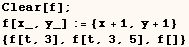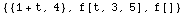Basic BlankSequence (__) usage

In the next example BlankSequence (__) is used and (x___) represents a sequence of one or more arguments.  So in this case (f) is defined when it's given one or more argument, but undefined when given no arguments.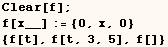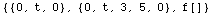Basic BlankNullSequence (___) usage

In the next example BlankNullSequence(___) is used and (x___) refers to a sequence of any number of arguments, or an empty set of arguments.  In this case f[] returns {0,0} while (f) was undefined in the previous example.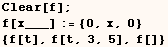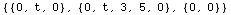Use of Blanks without naming a variable

If a function doesn't use some of the arguments, it's more efficient to not name them.
In the following example I define a function that returns True when given any single argument.  Often times we will give a function other definitions to use in other cases, and make it use a catch all definition such as this in all other cases.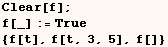In the next cell (f) returns True when given one or more argument.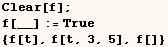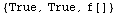In the next cell (f) returns True when given any number of arguments or no arguments at all.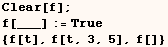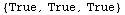A more common use of an unamed BlankNullSequence is in the next cell.  In this case (f) is defined when it gets two or more arguments.  The first argument goes with (x_), the second argument goes with (y_), and all other arguments go with (___).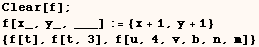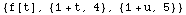Use of named Blanks with a specified head

In the next example (f) is only defined when given two arguments that are symbols.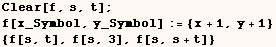In the next example (x__Real) represents a sequence of one or more arguments that are symbols.  If any argument of (f) is not a symbol, (f) is not defined.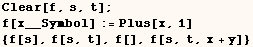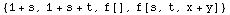In the next example BlankNullSequence(___) is used and (x___Symbol) refers to a sequence of any number of arguments with the head symbol, or an empty set of arguments.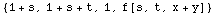Use of unamed Blanks with a specified head

In the next few examples there is no advantage in naming the arguments, and the definition evaluated faster than one where the argument is named.  In the next cell (f) returns True when given a single argument with the Head Real.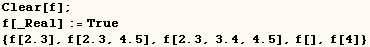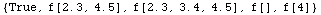In the next cell (f) returns True when given one or more argument and all the arguments have the Head Real.In the next cell (f) returns True when given any number of arguments or no arguments at all, but only when all arguments given have the Head Real.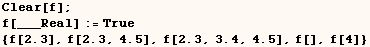Created by Mathematica  (May 16, 2004)